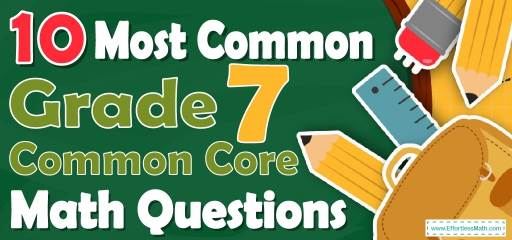# 10 Most Common 7th Grade Common Core Math QuestionsMake sure to follow some of the related links at the bottom of this post to get a better idea of what kind of mathematics questions students need to practice.

## 10 Sample 7th Grade Common Core Math Practice Questions

1- What is the median of these numbers? $$2, 28, 28, 19, 67, 44, 35$$

A. 19

B. 28

C. 44

D. 35

2- Last week 24,000 fans attended a football match. This week three times as many bought tickets, but one-sixth of them canceled their tickets. How many are attending this week?

A. 48000

B. 54000

C. 60000

D. 72000

3- The following trapezoids are similar. What is the value of $$x$$?

A. 7

B. 8

C. 18

D. 45

4- If $$x=- 8$$, which equation is true?

A. $$x(2x-4)=120$$

B. $$8 (4-x)=96$$

C.$$2 (4x+6)=79$$

D. $$6x-2=-46$$

5- In a bag of small balls $$\frac{1}{3}$$ are black, $$\frac{1}{6}$$ are white, $$\frac{1}{4}$$ are red and the remaining 12 blue. How many balls are white?

A. 8

B. 12

C. 16

D. 24

6- A boat sails 40 miles south and then 30 miles east. How far is the boat from its start point?

A. 45

B. 50

C. 60

D. 70

7- Sophia purchased a sofa for $530.40. The sofa is regularly priced at$624. What was the percent discount Sophia received on the sofa?

A. $$12\%$$

B. $$15\%$$

C. $$20\%$$

D. $$25\%$$

8- The score of Emma was half as that of Ava and the score of Mia was twice that of Ava. If the score of Mia was 60, what is the score of Emma?

A. 12

B. 15

C. 20

D. 30

9- A bag contains 18 balls: two green, five black, eight blue, a brown, a red, and one white. If 17 balls are removed from the bag at random, what is the probability that a brown ball has been removed?

A. $$\frac{1}{9}$$

B. $$\frac{1}{6}$$

C. $$\frac{16}{17}$$

D. $$\frac{17}{18}$$

10- A rope weighs 600 grams per meter in length. What is the weight in kilograms of 12.2 meters of this rope? (1 kilograms = 1000 grams)

A. 0.0732

B. 0.732

C. 7.32

D. 7320

## Best 7th Grade Common Core Math Prep Resource for 2022

1- B
Write the numbers in order:
$$2, 19, 28, 28, 35, 44, 67$$
Since we have 7 numbers (7 is odd), then the median is the number in the middle, which is 28.

2- C
Three times 24,000 is 72,000. One-sixth of them canceled their tickets.
One sixth of 72,000 equals 12,000 $$(\frac{1}{6}) × 72000 = 12000$$.
60,000 $$72000 – 12000 = 60000$$ fans are attending this week

3- A
It’s needed to have a ratio to find value of $$x$$.
$$\frac{45}{40}=\frac{2x+4}{16}⇒ 40(2x+4)=45×16 ⇒ x=7$$

4- C
$$8 (4-(-8))=96$$

5- A
$$\frac{1}{3}x + \frac{1}{6}x + \frac{1}{4}x + 12= x$$
$$(\frac{1}{3} + \frac{1}{6} + \frac{1}{4}) x+ 12= x$$
$$(\frac{9}{12})x+ 12 = x$$
$$x = 48$$
In a bag of small balls $$\frac{1}{6}$$ are white then: $$\frac{48}{6} = 8$$

6- B
Use the information provided in the question to draw the shape.
Use Pythagorean Theorem: $$a^2 + b^2 = c^2$$
$$40^2 + 30^2 = c^2 ⇒ 1600 + 900 = c^2 ⇒ 2500 = c^2 ⇒ c = 50$$

7- B
The question is this: 530.40 is what percent of 624?
Use percent formula:
$$part = \frac{percent}{100}× whole$$
$$530.40= \frac{percent}{100}× 624 ⇒ 530.40 = \frac{percent ×624}{100}⇒53040 = percent ×624$$
$$⇒percent = \frac{53040}{624}= 85$$
530.40 is $$85 \%$$ of 624. Therefore, the discount is: $$100\% – 85\% = 15\%$$

8- B
If the score of Mia was 60, therefore the score of Ava is 30. Since the score of Emma was half as that of Ava, therefore, the score of Emma is 15.

9- D
If 17 balls are removed from the bag at random, there will be one ball in the bag.
The probability of choosing a brown ball is 1 out of 18. Therefore, the probability of not choosing a brown ball is 17 out of 18 and the probability of having not a brown ball after removing 17 balls is the same.

10- C
The weight of 12.2 meters of this rope is: $$12.2 × 600 \space g = 7320 \space g$$
$$1\space kg = 1000 \space g$$ therefore,
$$7320 \space g ÷ 1000 = 7.32 \space kg$$

Looking for the best resource to help you succeed on the Grade 7 Common Core Math test?

## The Best Books to Ace 7th Grade Common CoreMath Test

### What people say about "10 Most Common 7th Grade Common Core Math Questions - Effortless Math: We Help Students Learn to LOVE Mathematics"?

No one replied yet.

X
27% OFF

Limited time only!

Save Over 27%

SAVE $5 It was$18.99 now it is \$13.99